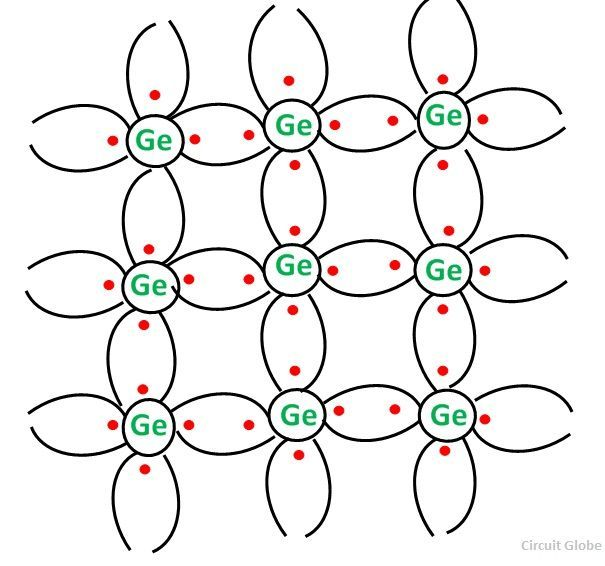# n Type Semiconductor

When a small amount of Pentavalent impurity is added to a pure semiconductor providing a large number of free electrons in it, the extrinsic semiconductor thus formed is known as n-Type Semiconductor. The conduction in the n-type semiconductor is because of the free electrons denoted by the pentavalent impurity atoms.

These electrons are the excess free electrons with regards to the number of free electrons required to fill the covalent bonds in the semiconductors.

Contents:

The addition of Pentavalent impurities such as arsenic and antimony provides a large number of free electrons in the semiconductor crystal. Such impurities which produce n-type semiconductors are known as Donor Impurities.

They are called a donor impurity because each atom of them donates one free electron crystal.When a few Pentavalent impurities such as Arsenic whose atomic number is 33, which is categorised as 2, 8, 15 and 5. It has five valence electrons, which is added to germanium crystal. Each atom of the impurity fits in four germanium atoms as shown in the figure above.

Hence, each Arsenic atom provides one free electron in the Germanium crystal. Since an extremely small amount of arsenic, impurity has a large number of atoms; it provides millions of free electrons for conduction.

## Energy Diagram of n-Type Semiconductor

The Energy level diagram of the n-type semiconductor is shown in the figure below:A large number of free electrons are available in the conduction band because of the addition of the Pentavalent impurity. These electrons are free electrons which did not fit in the covalent bonds of the crystal. However, a minute quantity of free electrons is available in the conduction band forming hole-electron pairs.

The following points are important in case of the n-type semiconductor:

• The addition of Pentavalent impurity results in a large number of free electrons.
• When thermal energy at room temperature is imparted to the semiconductor, a hole-electron pair is generated and as a result, a minute quantity of free electrons are available. These electrons leave behind holes in the valence band.
• Here n stands for negative material as the number of free electrons provided by the Pentavalent impurity is greater than the number of holes.

## Conduction Through n-Type Semiconductor

In the n-type semiconductor, a large number of free electrons are available in the conduction band which are donated by the impurity atoms. The figure below shows the conduction process of an n-type semiconductor:When a potential difference is applied across this type of semiconductor, the free electrons are directed towards the positive terminals. It carries an electric current. As the flow of current through the crystal is constituted by free electrons which are carriers of the negative charge, therefore, this type of conductivity is known as negative or n-type conductivity.

The electron-hole pairs are formed at room temperature. These holes which are available in small quantity in the valence band also consists of a small amount of current. For practical purposes, this current is neglected.

Also See: p-type Semiconductor